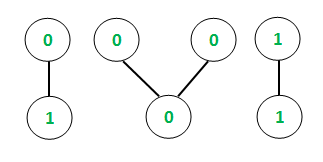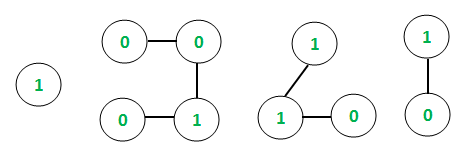# Hexadecimal equivalents in Binary Valued Graph

Given a binary valued undirected graph with V vertices and E edges, the task is to find the hexadecimal equivalents of all the connected components of the graph. A binary valued graph can be considered as having only binary numbers (0 or 1) as the vertex values.

Examples:

Input: E = 4, V = 7Output:
Chain = 0 1      Hexadecimal equivalent = 1
Chain = 0 0 0      Hexadecimal equivalent = 0
Chain = 1 1      Hexadecimal equivalent = 3
Explanation:
In case of the first connected component, the binary chain is [0, 1]
Hence, the binary string = “01” and binary number = 01
So, the hexadecimal equivalent = 1

Input: E = 6, V = 10Output:
Chain = 1      Hexadecimal equivalent = 1
Chain = 0 0 1 0      Hexadecimal equivalent = 2
Chain = 1 1 0      Hexadecimal equivalent = 6
Chain = 1 0      Hexadecimal equivalent = 2

Approach: The idea is to use Depth First Search Traversal to keep track of the connected components in the undirected graph as explained in this article. For each connected component, the binary string is displayed and the equivalent hexadecimal value is calculated from the binary value as explained in this article and printed.

Below is the implementation of the above approach:

## C++

 `// C++ implementation to find  ` `// hexadecimal equivalents of  ` `// all connected components  ` `#include ` `using` `namespace` `std; ` ` `  `// Function to traverse the undirected  ` `// graph using the Depth first traversal ` `void` `depthFirst(``int` `v, ` `                ``vector<``int``> graph[], ` `                ``vector<``bool``>& visited, ` `                ``vector<``int``>& storeChain) ` `{ ` `    ``// Marking the visited ` `    ``// vertex as true ` `    ``visited[v] = ``true``; ` ` `  `    ``// Store the connected chain ` `    ``storeChain.push_back(v); ` ` `  `    ``for` `(``auto` `i : graph[v]) { ` `        ``if` `(visited[i] == ``false``) { ` ` `  `            ``// Recursive call to ` `            ``// the DFS algorithm ` `            ``depthFirst(i, graph, ` `                       ``visited, ` `                       ``storeChain); ` `        ``} ` `    ``} ` `} ` ` `  `// Function to create map between binary ` `// number and its equivalent hexadecimal ` `void` `createMap(unordered_map* um) ` `{ ` ` `  `    ``(*um)[``"0000"``] = ``'0'``; ` `    ``(*um)[``"0001"``] = ``'1'``; ` `    ``(*um)[``"0010"``] = ``'2'``; ` `    ``(*um)[``"0011"``] = ``'3'``; ` `    ``(*um)[``"0100"``] = ``'4'``; ` `    ``(*um)[``"0101"``] = ``'5'``; ` `    ``(*um)[``"0110"``] = ``'6'``; ` `    ``(*um)[``"0111"``] = ``'7'``; ` `    ``(*um)[``"1000"``] = ``'8'``; ` `    ``(*um)[``"1001"``] = ``'9'``; ` `    ``(*um)[``"1010"``] = ``'A'``; ` `    ``(*um)[``"1011"``] = ``'B'``; ` `    ``(*um)[``"1100"``] = ``'C'``; ` `    ``(*um)[``"1101"``] = ``'D'``; ` `    ``(*um)[``"1110"``] = ``'E'``; ` `    ``(*um)[``"1111"``] = ``'F'``; ` `} ` ` `  `// Function to return hexadecimal ` `// equivalent of each connected ` `// component ` `string hexaDecimal(string bin) ` `{ ` `    ``int` `l = bin.size(); ` `    ``int` `t = bin.find_first_of(``'.'``); ` ` `  `    ``// Length of string before '.' ` `    ``int` `len_left = t != -1 ? t : l; ` ` `  `    ``// Add min 0's in the beginning ` `    ``// to make left substring length ` `    ``// divisible by 4 ` `    ``for` `(``int` `i = 1; ` `         ``i <= (4 - len_left % 4) % 4; ` `         ``i++) ` ` `  `        ``bin = ``'0'` `+ bin; ` ` `  `    ``// If decimal point exists ` `    ``if` `(t != -1) { ` ` `  `        ``// Length of string after '.' ` `        ``int` `len_right = l - len_left - 1; ` ` `  `        ``// Add min 0's in the end to ` `        ``// make right substring length ` `        ``// divisible by 4 ` `        ``for` `(``int` `i = 1; ` `             ``i <= (4 - len_right % 4) % 4; ` `             ``i++) ` ` `  `            ``bin = bin + ``'0'``; ` `    ``} ` ` `  `    ``// Create map between binary ` `    ``// and its equivalent hex code ` `    ``unordered_map ` `        ``bin_hex_map; ` `    ``createMap(&bin_hex_map); ` ` `  `    ``int` `i = 0; ` `    ``string hex = ``""``; ` ` `  `    ``while` `(1) { ` ` `  `        ``// Extract from left, ` `        ``// substring of size 4 and add ` `        ``// its hex code ` `        ``hex += bin_hex_map[bin.substr(i, 4)]; ` `        ``i += 4; ` ` `  `        ``if` `(i == bin.size()) ` `            ``break``; ` ` `  `        ``// If '.' is encountered add it ` `        ``// to result ` `        ``if` `(bin.at(i) == ``'.'``) { ` ` `  `            ``hex += ``'.'``; ` `            ``i++; ` `        ``} ` `    ``} ` ` `  `    ``// Required hexadecimal number ` `    ``return` `hex; ` `} ` ` `  `// Function to find the hexadecimal ` `// equivalents of all connected ` `// components ` `void` `hexValue( ` `    ``vector<``int``> graph[], ` `    ``int` `vertices, ` `    ``vector<``int``> values) ` `{ ` ` `  `    ``// Initializing boolean array ` `    ``// to mark visited vertices ` `    ``vector<``bool``> visited(10001, ` `                         ``false``); ` ` `  `    ``// Following loop invokes ` `    ``// DFS algorithm ` `    ``for` `(``int` `i = 1; i <= vertices; ` `         ``i++) { ` ` `  `        ``if` `(visited[i] == ``false``) { ` ` `  `            ``// Variable to hold ` `            ``// temporary length ` `            ``int` `sizeChain; ` ` `  `            ``// Container to store ` `            ``// each chain ` `            ``vector<``int``> storeChain; ` ` `  `            ``// DFS algorithm ` `            ``depthFirst(i, graph, ` `                       ``visited, ` `                       ``storeChain); ` ` `  `            ``// Variable to hold each ` `            ``// chain size ` `            ``sizeChain = storeChain.size(); ` ` `  `            ``// Container to store ` `            ``// values of vertices of ` `            ``// individual chains ` `            ``int` `chainValues[sizeChain + 1]; ` ` `  `            ``// Storing the values of ` `            ``// each chain ` `            ``for` `(``int` `i = 0; ` `                 ``i < sizeChain; i++) { ` ` `  `                ``int` `temp = values[storeChain[i] - 1]; ` `                ``chainValues[i] = temp; ` `            ``} ` ` `  `            ``// Printing binary chain ` `            ``cout << ``"Chain = "``; ` ` `  `            ``for` `(``int` `i = 0; ` `                 ``i < sizeChain; i++) { ` ` `  `                ``cout << chainValues[i] ` `                     ``<< ``" "``; ` `            ``} ` `            ``cout << ``"\t"``; ` ` `  `            ``// Converting the array ` `            ``// with vertex ` `            ``// values to a binary string ` `            ``// using string stream ` `            ``stringstream ss; ` `            ``ss << chainValues; ` `            ``string s = ss.str(); ` ` `  `            ``for` `(``int` `i = 1; ` `                 ``i < sizeChain; i++) { ` ` `  `                ``stringstream ss1; ` `                ``ss1 << chainValues[i]; ` `                ``string s1 = ss1.str(); ` `                ``s.append(s1); ` `            ``} ` ` `  `            ``// Printing the hexadecimal ` `            ``// values ` `            ``cout << ``"Hexadecimal "` `                 ``<< ``"equivalent = "``; ` `            ``cout << hexaDecimal(s) ` `                 ``<< endl; ` `        ``} ` `    ``} ` `} ` ` `  `// Driver Program ` `int` `main() ` `{ ` `    ``// Initializing graph in the ` `    ``// form of adjacency list ` `    ``vector<``int``> graph; ` ` `  `    ``// Defining the number of ` `    ``// edges and vertices ` `    ``int` `E, V; ` `    ``E = 4; ` `    ``V = 7; ` ` `  `    ``// Assigning the values ` `    ``// for each vertex of the ` `    ``// undirected graph ` `    ``vector<``int``> values; ` `    ``values.push_back(0); ` `    ``values.push_back(1); ` `    ``values.push_back(1); ` `    ``values.push_back(1); ` `    ``values.push_back(0); ` `    ``values.push_back(1); ` `    ``values.push_back(1); ` ` `  `    ``// Constructing the ` `    ``// undirected graph ` `    ``graph.push_back(2); ` `    ``graph.push_back(1); ` `    ``graph.push_back(4); ` `    ``graph.push_back(3); ` `    ``graph.push_back(5); ` `    ``graph.push_back(4); ` `    ``graph.push_back(5); ` `    ``graph.push_back(6); ` `    ``graph.push_back(7); ` `    ``graph.push_back(6); ` ` `  `    ``hexValue(graph, V, values); ` `    ``return` `0; ` `} `

Output:

```Chain = 0 1
Chain = 1 1 0 1 1
```

Time Complexity: O(V2)
The DFS algorithm requires O(V + E) complexity, where V, E are the vertices and edges of the undirected graph. Further, the hexadecimal equivalent is obtained at each iteration which requires an additional O(V) complexity to compute. Hence, the overall complexity is O(V2).My Personal Notes arrow_drop_upCheck out this Author's contributed articles.

If you like GeeksforGeeks and would like to contribute, you can also write an article using contribute.geeksforgeeks.org or mail your article to contribute@geeksforgeeks.org. See your article appearing on the GeeksforGeeks main page and help other Geeks.

Please Improve this article if you find anything incorrect by clicking on the "Improve Article" button below.